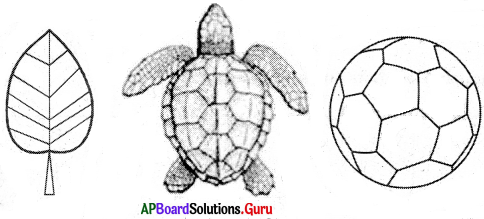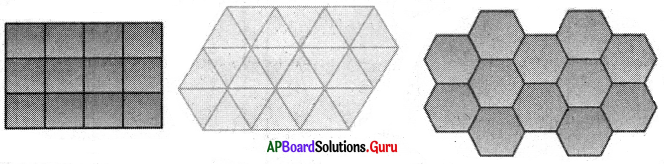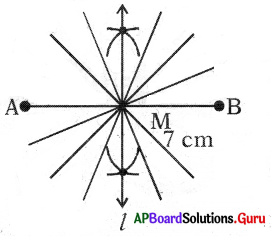SCERT AP 7th Class Maths Solutions Pdf Chapter 12 Symmetry Unit Exercise Questions and Answers.

## AP State Syllabus 7th Class Maths Solutions 12th Lesson Symmetry Unit Exercise

Question 1.
Fill in the blanks.
(i) Afigure has _______ symmetry if there is a line about which the figure may be folded so that the two parts of the figure coincide.
Line

(ii) A regular pentagon has _______ line of symmetry.
Five

(iii) A figure has _______ symmetry if after a rotation through a certain angle. The figure looks exactly the same.
Rotational

(iv) A _______ triangle has no lines of symmetry.
Scalene

(v) Each regular polygon has as many lines of symmetry as it has _______
Number of sides

(vi) The concept of line symmetry is closely related to _______ reflection.
Mirror

(vii) The quadrilateral that has four lines of symmetry and order-four rotational symmetry is a _______
Square

(viii) The angle of rotational symmetry for letter S is _______
180°

(ix) A line segment is symmetrical about its _______
Perpendicular bisector

(x) Station turns an object about a fixed point. The fixed point is called _______
Point of rotation(xi) Each of the letters H, N, S and Z has a rotational symmetry of order _______
2

(xii) The line of symmetry of an isosceles triangle is it’s _______ from the vertex having the equal sides.
Median/altitude/angular bisector

Question 2.
Cut the capital letters of English and paste them in your note book. Draw possible number of lines of symmetry for each of the letter.
(i) How many letters have no line of symmetry? What are thpy?
10. They are F, G, J, L, N, P, Q, R, S, Z.

(ii) How many letters have one line of symmetry? What are they?
12. They are A, B, C, D, E, K, M, T, U, V, W, Y.

(iii) How many letters have two lines of symmetry? What are they?
3. They are H, I, X.

(iv) How many letters have more than two lines of symmetry? What are they?
1. That is 0.(v) Which of them have rotational symmetry? What are they?
4. They are H, I, 0, X.

(vi) Which of them have point symmetry? What are they?
7. They are H, I, N, 0, S, X, Z.

Question 3.
Draw some natural objects which have at least one line of symmetry.Question 4.
Draw three tessellations and expose the basic shapes used on your tessellation.4. So, the line segment $$\widehat{\mathrm{AB}}$$ has infinite lines.